Learning Library

Exercises

# Measurement and Data 4

Representing data on graphs makes math visual and involves some creativity and design. Plotting data using fractional units takes their learning one step further. Also in this unit, students learn to measure using both traditional units (like inches, feet and yards) and metric units, and how to convert measurements within each system. The concept of measurement gets two dimensional when students explore measuring and working with angles.
This lesson includes 5 printable learning activities.

## Collecting and Organizing Data 2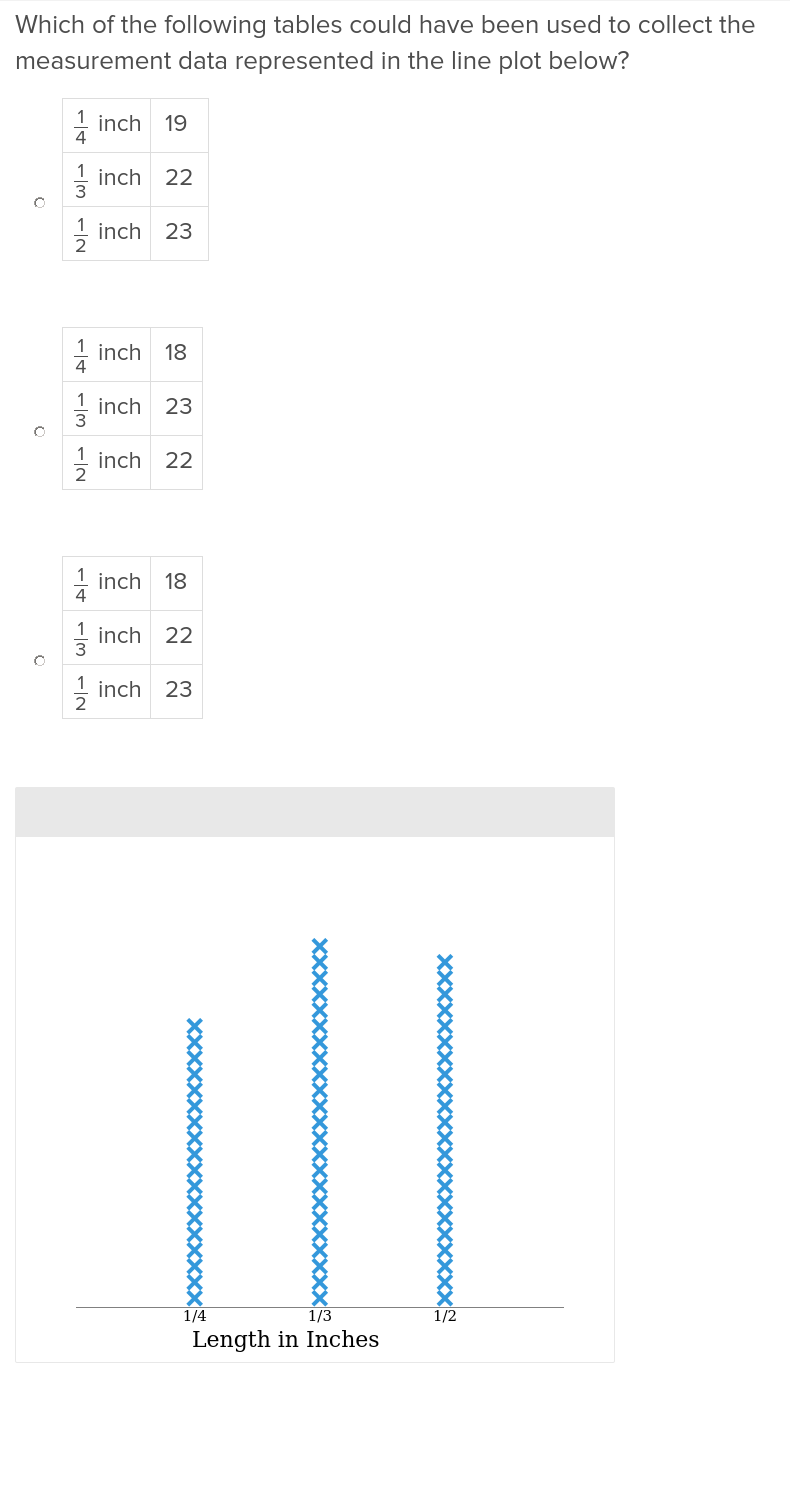Your students will grow adept at collecting and organizing data around them with this engaging exercise.

## Probability and Statistics 2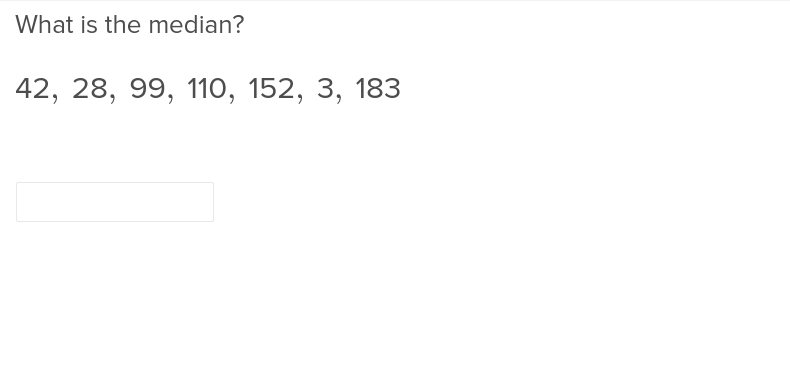Continue the probability and statistics fun with this involving exercise that shows students how to use data to make predictions.

## Line Plots and Unit Fractions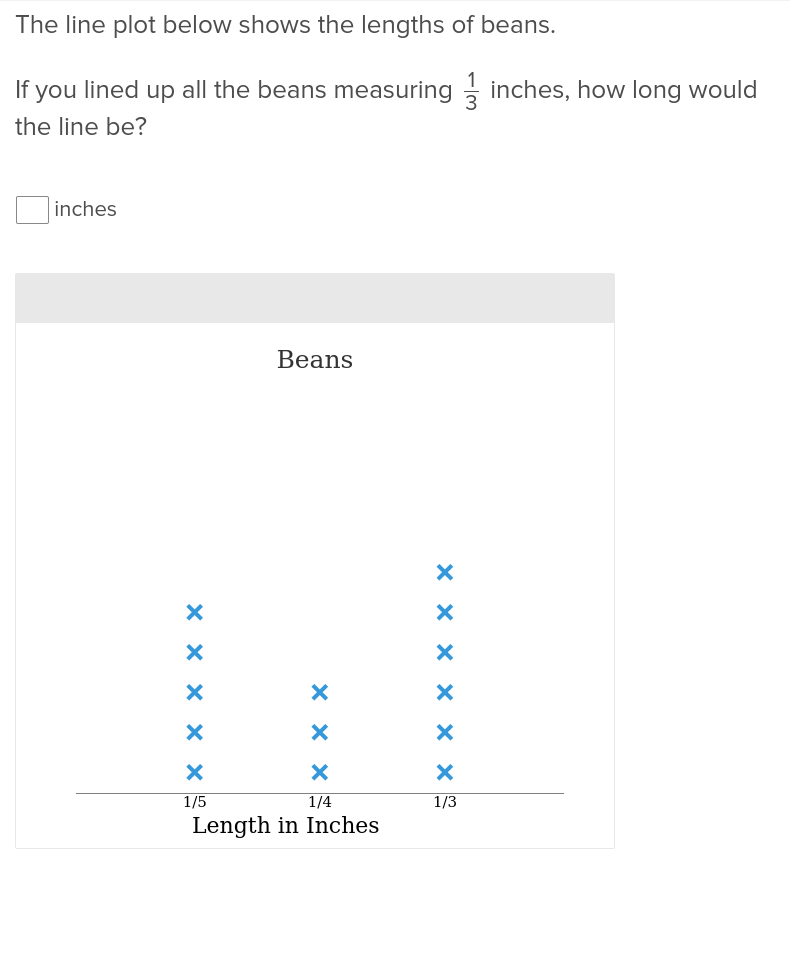Understanding unit fractions is a lot easier with this exercise that lays out all out on line plots.

## Systems of Measurement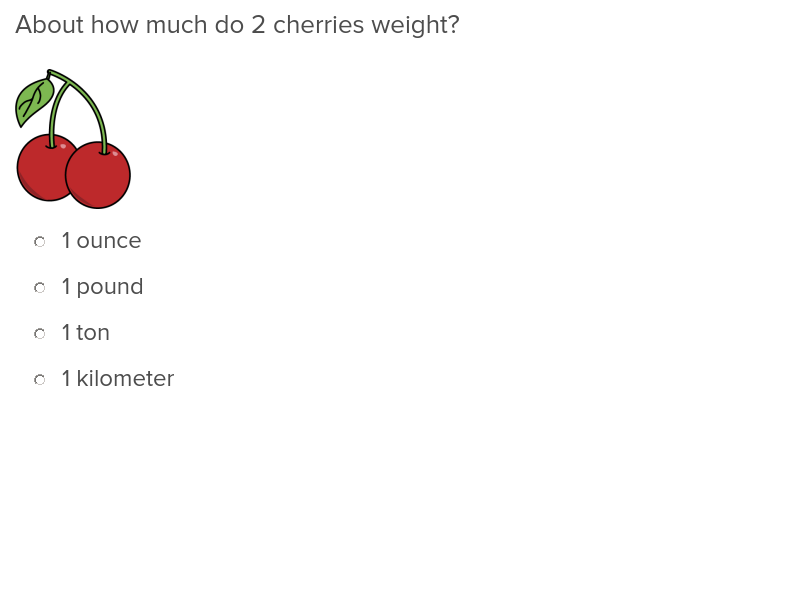Students will broaden their horizons with this exercise that teaches about different systems of measurement.

## Fractions and Metric Units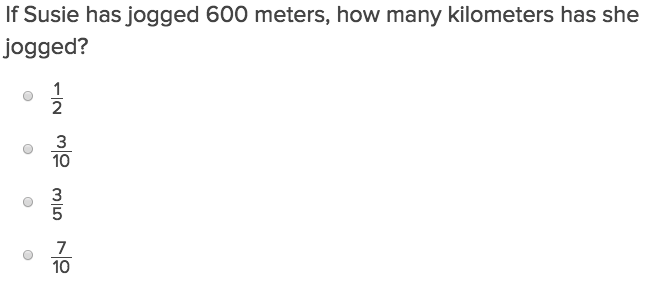Fractions and Metric Units will help students practice this key fourth grade skill.

## Measurement and Equivalence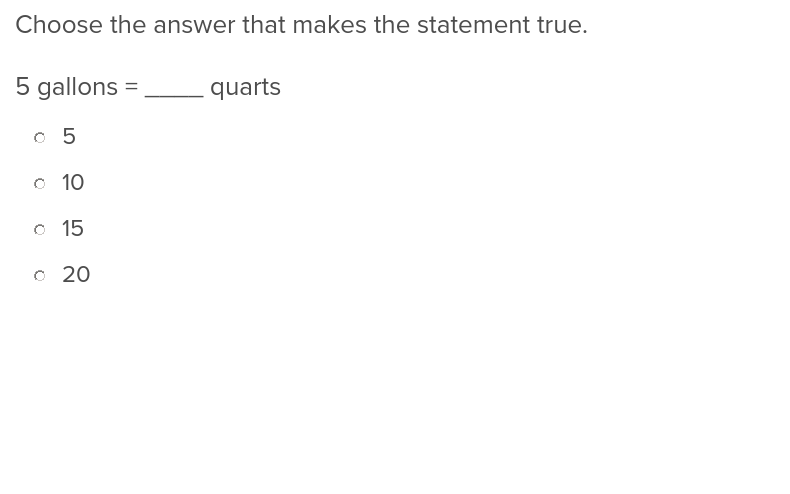Show students how to measure and find the equivalent in another system with this exercise.

## Measurement and Graphing 2Teach students how to take measurements and plot them on graphs with this easy to use exercise.

## Circus Angle Measurement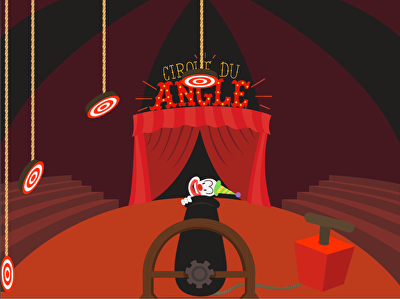Come one, come all! This circus-themed geometry game provides a unique and entertaining way for fourth graders to practice estimating and measuring angles. In order to help the circus clowns hit the target, young mathematicians must identify the angle and aim the cannon correctly before sending Bozo flying across the tent. Perfect for students working on lines and angles, Circus Angle Measurement is a fun way to help kids grasp benchmark angles, such as 30, 90, or 180 degrees.

## Measuring Angles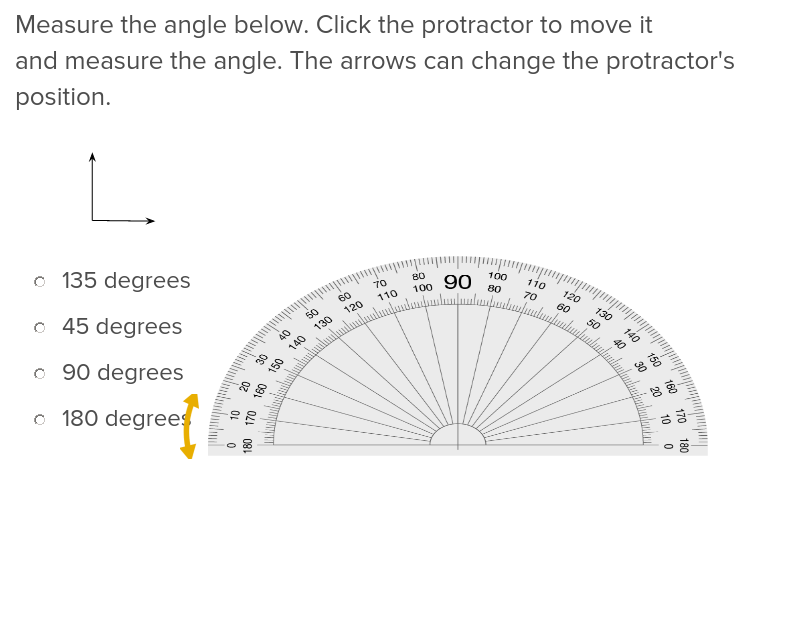Teach students how to measure angles without the confusion of a protractor with this easy to use exercise.

## Recognizing Angle Measure as Additive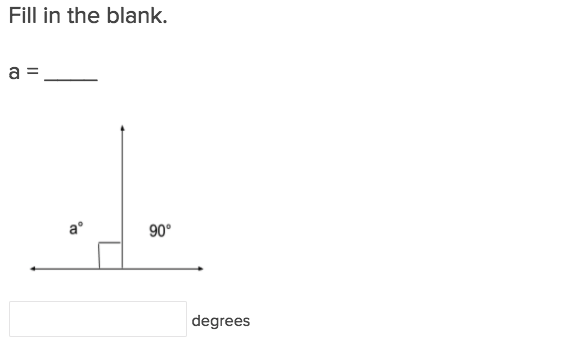Many students don’t realize they can use an angle measures as an additive until they have completed this exercise.

Create new collection

0

### New Collection>

0 items

What could we do to improve Education.com?